Courses

# Treatment of Sewage Notes | EduRev

## Civil Engineering (CE) : Treatment of Sewage Notes | EduRev

The document Treatment of Sewage Notes | EduRev is a part of the Civil Engineering (CE) Course Environmental Engineering.
All you need of Civil Engineering (CE) at this link: Civil Engineering (CE)

Sedimentation Tank

Settling Velocity

1.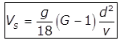for d < 0.1 mm
Where,
Vs = The velocity of the settlement of particle or settling velocity in m/sec.
d = The diameter of the particle in the meter.
G = Specific gravity of the particle.
v = Kinematic viscosity of water in m2/sec.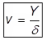Y = Dynamic viscosity
δ = Density

2.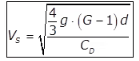where,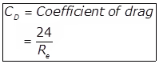→ For laminar flow
Re = Reynolds number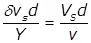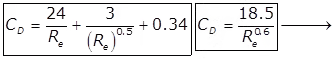for transition flow.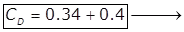for turbulent flow.

3.4. Newtons Equation for Turbulent Settling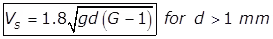5. Modified Hazen’s Equation for Transition Zone
(i)Where T = Temperature in oC.
(ii) Putting G = 2.65 for Inorganic Solids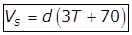(iii) Putting G = 1.2 for Organic Solids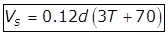Critical Scour Velocity in Constant Velocity Horizontal Flow

Grit Chamber (VH)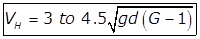Proportional Flow Weir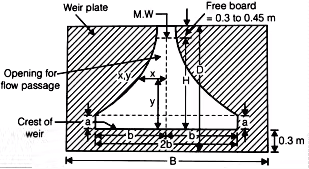Where,
B = Width of the channel.
Vh = Horizontal flow velocity.
Cd = Coefficient of discharge.
x and y are coordinates on weir profile.

Parabolically or V-Shaped Grit Chamber Provided with a Parshall Flume

1. Parshall Flume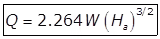Where,
W = Width of the throat in the meter.
Flow in (m3/sec) through Parshall flume.
Ha = Depth of flow in the upstream leg of a flume of one-third portion in the meter.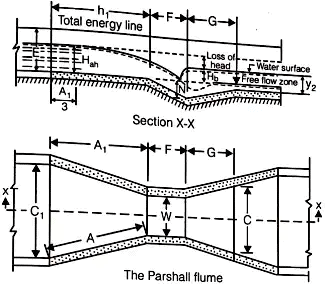2. Parabolic Grit ChannelWhere,
n = Discharge coefficients of the control section.
= 1.5 for partial flume.
= 1 for proportional flow weir.
(i) Aerated Grit Channels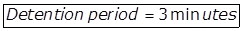(ii) Detritus Tank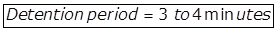Skimming Tank

1. Detention Period = 3 to 5 minutes.
2. Amount of compressed air required = 300 to 6000 m3 per million liters of sewage.
3. Surface Area,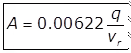Where,
q = Rate of flow of sewage in m3/day.
Vr = Min. rising velocity of greasy material to be removed in m/min
= 0.25 m/min mostly.

Vacuators

Vacuum Pressure = 0 to 25 cm of Hg
For 10 to 15 minutes.

1. Sedimentation Tank
(i) Overflow rate
= 40000 to 50000 lit/m2 day for plain sedimentation.
= 50000 to 60000 lit/m2 day for sedimentation with coagulation.
= 25000 to 35000 lit/m2 day for secondary sedimentation tank
(ii) Depth ~ 2.4 to 3.6 m.
(iii) Detention time = 1 to 2 hour.
(iv) width = 6.0 m
(v) Length = 4 to 5 times width.
(vi) Velocity of flow Vf = 0.3 m/min.
(vii)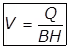Where,V = Flow velocity
B = Width of the Basin
H = Depth of sewage in the tank.
(viii)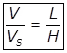(ix)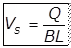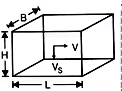2. Detention Time
(i)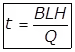For rectangular Tank
(ii)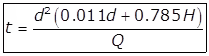for circular tank
Where
d = Dia of the tank
H = Vertical depth of wall or side depth
3.  Displacement Efficiency (η)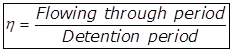Trickling Filter

1. Conventional Trickling Filter or Low Rate Trickling Filter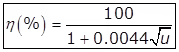Where,
η = The efficiency of the filter and its secondary clarifier, in terms of % of applied BOD
2. High Rate Trickling Filter
(i)Where, F = Recirculation factor
Recirculation ratio
(ii)Where,
Y = Total organic loading in kg/day applied to the filter i.e. the total BOD in kg.
V = Filter volume in Ha-m.
% efficiency of single-stage high rate trickling filter.
(iii)Where,
n' = Final efficiency in the two-stage filter.
Y' = Total BOD in the effluent from the first stage in kg/day.
F' = Recirculation factor for second stage filter
V' = Volume in second stage filter in ha-m.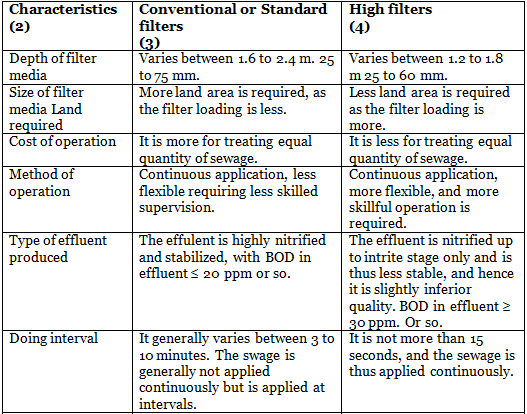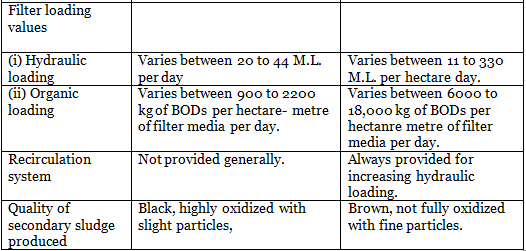Dunbar Filter
BOD removed = 85%
Sludge and its Moisture Content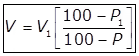The volume of sludge at moisture content P1%
The volume of sludge at moisture content P%

Sludge Digestion Tank

1. When the change during digestion is linear.
(i)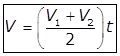Where,
The volume of digestion in m3.
Raw sludge added per day (m./day)
Equivalent digested sludge produced per day on completion of digestion, m3/day.
Digestion period in the day.
(ii)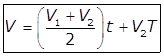with monsoon storage
Where,
T = Number of days for which digested sludge (V2) is stored (monsoon) storage)
2. When the change during digestion is parabolic.
(i)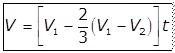without monsoon storage
(ii)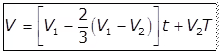without monsoon storage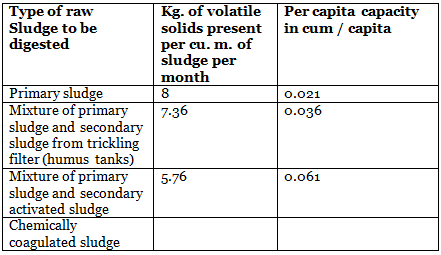Destruction and Removal Efficiency (DRE)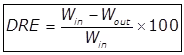Where,
Win = The mass fill rate of one POHC (Principal organic Hazardous constituent) in the waste stream.
Wout = Mass emission rate of the same POHC present in the exhaust emission prior to release to the atmosphere.

Aeration Tank (ASP)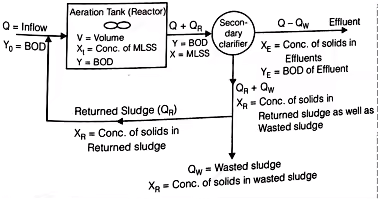1. Detention period,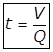Where
V = Volume of the tank in m3.
Q = Quantity of wastewater flow into the aeration tank excluding the quantity of recycled sludge (m3/day)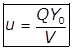Where,
QYo = Mass of BOD applied per day to the aeration tank through influent sewage in gm.
V = The volume of the aeration tank in m3.
Q = Sewage flows into the aeration tank in m3.
BOD5 in mg/lit (or gm/m3) of the influent sewage.

3.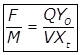Where,
F/M = Food (F) to Microorganism (M) ratio QYo = Daily BOD applied to the aeration system in gm.
Yo = 5 day BOD of the influent sewage in mg/lit.
Q = The flow of influent sewage in m3/day.
MLSS (Mixed liquor suspended solids) in mg/lit.
V = The volume of the Aeration Tank (lit).
M = XtV = Total microbial mass in the system in gm.
4. Sludge Age (θc)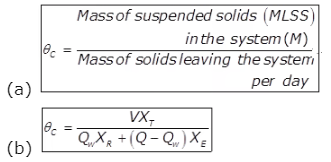Where,
XT = The concentration of solids in the influent of the Aeration Tank called the MLSS i.e. mixed liquor suspended solids in mg/lit.
V = Volume of Aerator
Qw = The volume of waste sludge per day
The concentration of solids in the returned sludge or in the wasted sludge (both being equal) in mg/lit.
Q = Sewage inflow per day.
XE = The concentration of solids in the effluent in mg/lit.
5. Sludge Volume Index (S.V.I)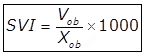Where,
Xab = Concentration of suspended solids in the mixed liquor in mg/lit.
Vab = Settled sludge volume in ml/lit.
S.V.I = Sludge volume index in ml/gm.
6. Sludge Recycle and Rate of Return Sludge
QR·XR = (Q + QR) x R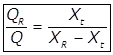Where,
QR = Sludge recirculation rate in m3/day.
Xt = MLSS in the aeration tank in mg/lit.
XR = MLSS in the returned or wasted sludge in mg/lit.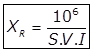S.V.I = Sludge volume index in ml/gm.
(i) Specific substrate utilization rate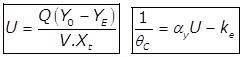αy = 1 for MLSS and 0.6 for MLVSS,  ke = 0.66
(ii) Oxygen Requirement of the Aeration Tank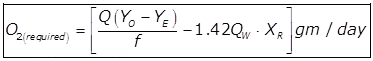Where,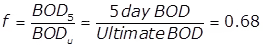(iii) Oxygen Transfer Capacity (N)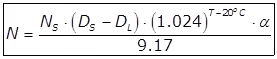Where,
N = Oxygen transferred under field conditions in kg O2/k.wh (Or MJ)
Ns = Oxygen transfer capacity under standard conditions in kg O2/kwh (or MJ)
Ds = Dissolved oxygen-saturation value for sewage at operating temperature.
DL = Operation D.O level in Areation tank usually 1 to 2 mg/lit.
T = Temperature in oC
α = Correction factor for oxygen transfer for sewage usually 0.8 to 0.85.

Oxidation Ponds

• Depth → 1.0 to 1.8 m.
• Detention period → 2 to 6 weeks.
• Under hot condition → 60 to 90 kg/ha/day.
Under cold conditions.
• Length to width ratio = 2
• Sludge Accumulation = 2 to 5 cm/year
• Minimum depth to be kept = 0.3 m.

For Inlet Pipe Design

Assume V = 0.9 m/s
Assume flow for 8 hrs.

For Outlet Pipe Design

Dia of outlet = 1.5 dia of the inlet pipe

Septic Tank

• Detention time = 12 to 36 hr.
• Sludge accumulation rate = 30 lit/cap/year.
• Sewage flow = 90 to 150 lit/capita/day.
• Cleaning period = 6 to 12 months
• Length to width ratio = 2 to 3 m.
• Depth = 1.2 to 1.8 m
•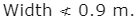• Free board = 0.3 m.
Volume of Septic Tank = (Sewage flow x Detention time) + (Sludge accumulation rate) x Clearning rate
Offer running on EduRev: Apply code STAYHOME200 to get INR 200 off on our premium plan EduRev Infinity!

## Environmental Engineering

14 videos|73 docs|70 tests

,

,

,

,

,

,

,

,

,

,

,

,

,

,

,

,

,

,

,

,

,

;